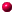"Inside  every small problem is a large problem struggling to get out."

RulesForumContributors[For contributors only]

## Thread and Full Text View

Ask a question about: Zero Sum Games
Respond to the question: find the probabilities and the game valu?

 11/27/2003 09:58 AM by leontios; find the probabilities and the game value of a payoff matrix with dual simplex
I there any program written in matlab to give as input the payoff matrix of a game and to give you as an output the corresponding probabilites of X and Y players and the game value? [Manage messages]